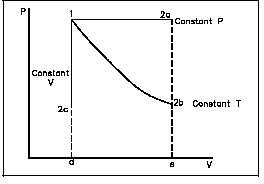# Boyle’s, Charles & Gay-Lussac’s Law from Ideal Gas Law

## The Ideal Gas Law and Avogadro’s Number

In terms of Avogadro’s number NA, we can write the number of moles n as:

1) n = N/NA

where N represents the total number of molecules in a gas and NA = 6.02 x 1023 molecules/mole. We found in part one that the ideal gas law may be written as:

2) PV = nRT

Substituting 1 into 2 yields:

3) PV = N/NART

We can simplify this equation even further with the use of Boltzmann’s contant. Boltzmann’s constant is defined as:

4) k = R/NA

which is 1.38 x 10-23 J/K in SI units. Then equation 3 becomes:

5) PV = NkT

This is another standard way of writing the ideal gas equation.

## Another Form

It is not always necessary to use the number of molecules or Boltzmann’s constant.In this case, the ideal gas law is also commonly written as:

6) P1V1 / T1 = P2V2 / T2

where P1,V1, and T1 are the original values of the gas, while P2,V2, and T2 represent its final values.

We can use equation 6 to derive Boyle’s, Charles’, and Gay-Lussac’s Laws. We do this by considering isothermal, isobaric, and isochoric thermodynamic processes.

## Derivation of Boyle’s Law

Isothermal

Isothermal means that the temperature is constant. When we do this, T1 = T2 = T, so:

7) P1V1 / T = P2V2 / T

The Ts cancel, and we are left with Boyle’s Law P1V1 = P2V2.

## Derivation of Charles’ Law

Isobaric

Isobaric means that the pressure is constant, so P1 = P2 = P, giving us:

8) PV1 / T1 = PV2 / T2

With the Ps canceling, we are left with Charles’ Law V1/T1 = V2/T2.

## Derivation of Gay-Lussac’s Law

Isochoric

Finally, isochoric means the volume is constant, such that V1 = V2 = V, and thus we have:

9) P1V / T1 = P2V / T2

The Vs cancel, giving us Gay-Lussac’s Law P1/T1 = P2/T2.

## References

Physics for Scientists and Engineers by Douglas Giancoli

Fundamentals of Physics by Halliday, Resnick, and Walker

Image Credits

Ideal gas law from www.EngineersEdge.com

## Resources

Comprehensive List of the Various Forms of the Ideal Gas Law

## This post is part of the series: Introduction to the Ideal Gas Law

This series gives an elementary, non-calculus based introduction to the ideal gas law, including an account of its origins and how to use it to derive Boyle’s, Charles’, and Gay-Lussac’s Laws.